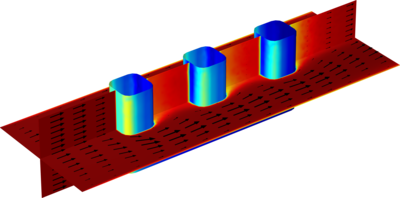# Galleria delle Applicazioni

## Wire Electrode

Application ID: 3471

The electrochemical cell shown in this model can be regarded as a unit cell of a larger wire-mesh electrode that is common in many industrial processes. One of the most important aspects in the design of electrochemical cells is the current density distributions in the electrolyte and electrodes. Non-uniform current density distributions can be detrimental for the operation of electrochemical processes. In many cases, the parts of an electrode that are subjected to a high current density can degrade at a faster rate. Knowledge of the current density distribution is necessary to optimize the utilization of the electrocatalysts that are typically comprised of expensive noble metals. Non-uniform deposition and consumption, unnecessarily high overvoltages, energy losses and possible unwanted side-reactions represent effects that should be minimized. This example simulates the primary, secondary, and tertiary current density distributions of an arbitrary electrochemical cell with wire electrodes. The current density distributions are investigated in succession so as to also demonstrate good working practices by gradually introducing complexity when modeling electrochemical cells.This model example illustrates applications of this type that would nominally be built using the following products: